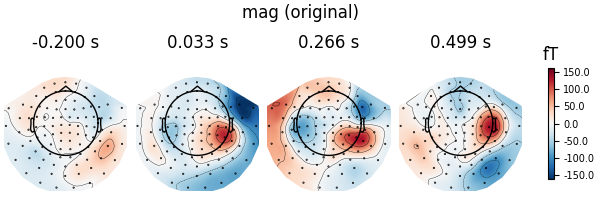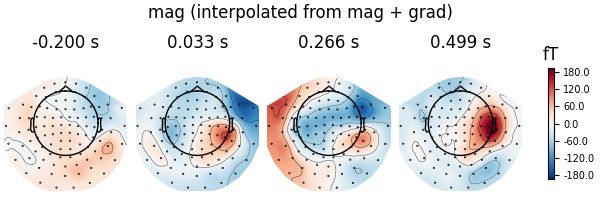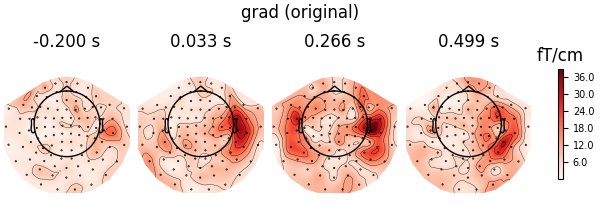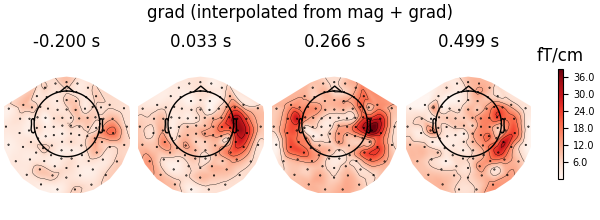# Remap MEG channel types#

In this example, MEG data are remapped from one channel type to another. This is useful to:

• run statistics from both magnetometers and gradiometers while working with a single type of channels.

```# Author: Mainak Jas <mainak.jas@telecom-paristech.fr>

```
```import mne
from mne.datasets import sample

print(__doc__)

data_path = sample.data_path()
meg_path = data_path / "MEG" / "sample"
fname = meg_path / "sample_audvis-ave.fif"
evoked = mne.read_evokeds(fname, condition="Left Auditory", baseline=(None, 0))
```
```Reading /home/circleci/mne_data/MNE-sample-data/MEG/sample/sample_audvis-ave.fif ...
Read a total of 4 projection items:
PCA-v1 (1 x 102) active
PCA-v2 (1 x 102) active
PCA-v3 (1 x 102) active
Average EEG reference (1 x 60) active
Found the data of interest:
t =    -199.80 ...     499.49 ms (Left Auditory)
0 CTF compensation matrices available
nave = 55 - aspect type = 100
Projections have already been applied. Setting proj attribute to True.
Applying baseline correction (mode: mean)
```

First, let’s call remap gradiometers to magnometers, and plot the original and remapped topomaps of the magnetometers.

```# go from grad + mag to mag and plot original mag
virt_evoked = evoked.as_type("mag")
fig = evoked.plot_topomap(ch_type="mag")
fig.suptitle("mag (original)")
``````Computing dot products for 305 MEG channels...
Computing cross products for 305 → 102 MEG channels...
Preparing the mapping matrix...
Truncating at 210/305 components to omit less than 0.0001 (9.9e-05)
```
```# plot interpolated grad + mag
fig = virt_evoked.plot_topomap(ch_type="mag")
fig.suptitle("mag (interpolated from mag + grad)")
```Now, we remap magnometers to gradiometers, and plot the original and remapped topomaps of the gradiometers

```# go from grad + mag to grad and plot original grad
``````Computing dot products for 305 MEG channels...
Computing cross products for 305 → 203 MEG channels...
Preparing the mapping matrix...
Truncating at 210/305 components to omit less than 0.0001 (9.9e-05)
```
```# plot interpolated grad + mag